# Dodecagons - polygons with 12 sides

By Martin McBride, 2022-10-10
Tags: dodecagon 12 sided shape regular polygon irregular polygon
Categories: gcse geometryA dodecagon is a flat shape with 12 straight sides.

## Regular and irregular dodecagons

A regular dodecagon is a 12-sided shape where every side is the same length and every corner has the same angle. All regular dodecagons have the same shape, like this: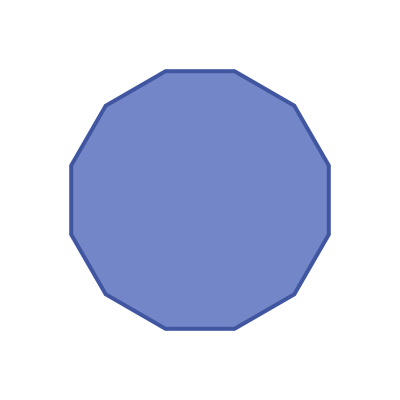An irregular dodecagon is a 12 sides shape where not every side and angle are equal. There are many different irregular dodecagon shapes, here is an example: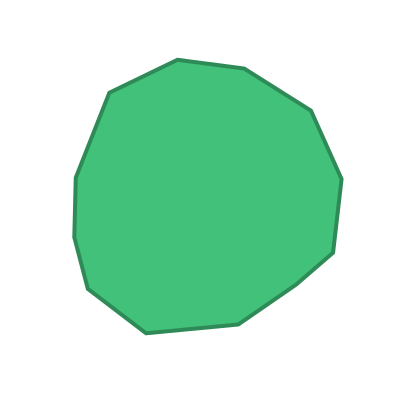## Name

The name dodecagon is a combination of the words dodeca (Greek meaning twelve) and gonia (Greek meaning corner).

Dodecagons are sometimes called 12-gons.

## Interior angles

The interior angles of a dodecagon are shown here: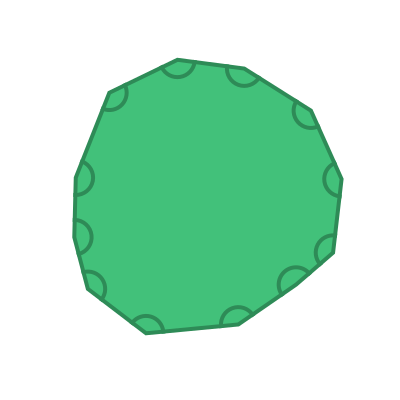The sum of these 12 angles is given by the formula:

sum of interior angles = (n - 2) x 180


Where n is the number of sides. In this case, the number of sides n is 12, so the sum of the interior angles is:

(12 - 2) x 180 = 1800 degrees


For a regular dodecagon, all the interior angles are equal: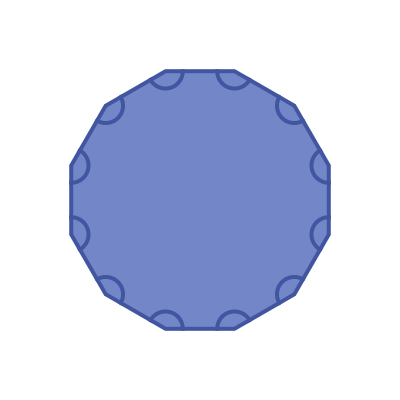This means that the interior angle of a regular dodecagon is:

1800 / 12 =  150 degrees


## Exterior angles

The exterior angles of a dodecagon are shown here: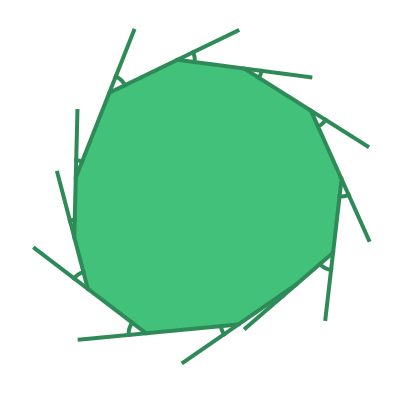The sum of the exterior angles of any polygon is 360 degrees.

For a regular dodecagon, all the interior angles are equal: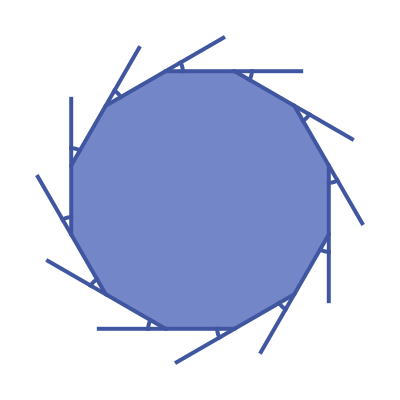This means that the exterior angle of a regular dodecagon is:

360 / 12 =  30 degrees


## Symmetry of regular dodecagons

A regular dodecagon has 12 lines of symmetry. This means that it can be reflected over each of the 12 grey lines shown here: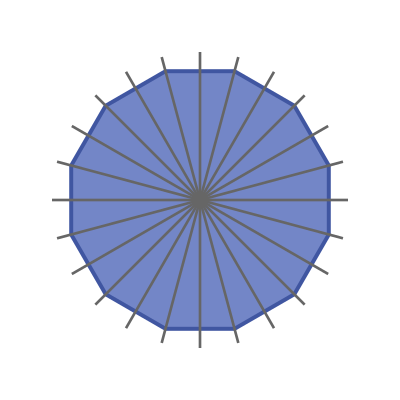A regular dodecagon has rotational symmetry of order 12. This means that if it rotated about its centre by a 12th of a full turn, it will map onto itself: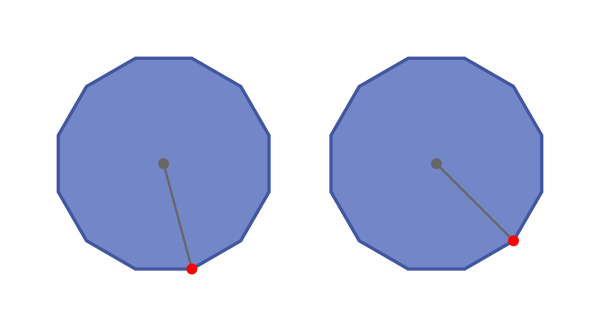## Real life examples

A number of coins around the world are regular dodecagons, including the British pound.